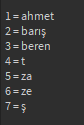# Sorting string keys in custom alphabet order

I have a table with strings and I need to sort in alphabetical order but some letters are not correct
so I made table (Turkish alphabet with some english letters at the end)

``````letters={"a","b","c","ç","d","e","g","h","ğ","h","ı","i","j","k","l","m","n","o","ö","p","r","s","ş","t","u","ü","v","y","z","x","w","q"}
``````

I need to sort alphabetically with this order

``````local ListToSort = {
= "beren",
= "barış",
= "ahmet",
= "ze",
= "za",
= "ş",
= "t",
}
``````
1 Like

Here you go:

``````local letters = {"a","b","c","ç","d","e","g","h","ğ","h","ı","i","j","k","l","m","n","o","ö","p","r","s","ş","t","u","ü","v","y","z","x","w","q"}

local listToSort = {"beren", "barış","ahmet", "ze", "za", "ş", "t"}

table.sort(listToSort, function(a, b)
return a < b
end)

for i, v in ipairs(listToSort) do
print(i .. " = " .. v)
end
``````

Output:you didnt use letters table in sorting. Your code is sorting with english alphabet

expected output is

``````ahmet
barış
beren
ş
t
za
ze
``````
2 Likes